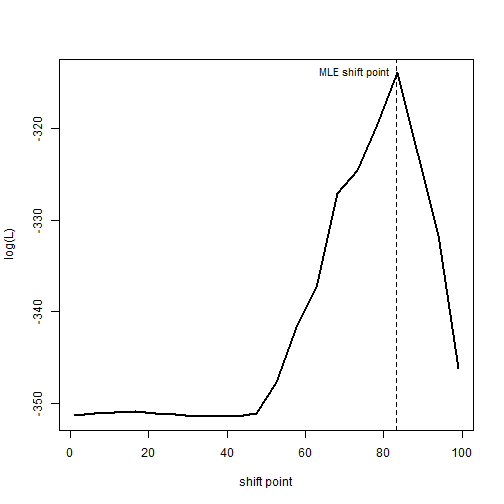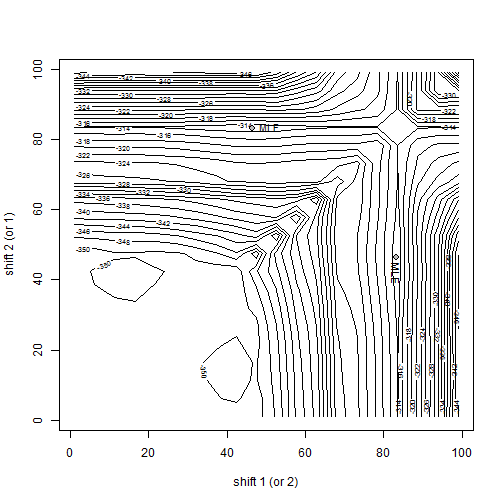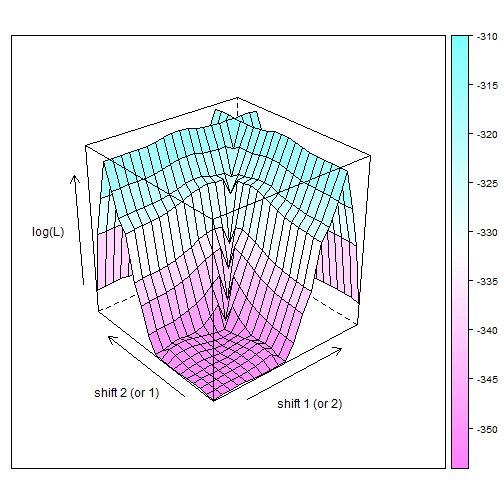## Tuesday, August 16, 2016

### Function to plot the likelihood surface for `rateshift` method

I just added a new function to visualize the likelihood surface in one & two dimensions for the phytools function `rateshift`.

The function `rateshift` uses likelihood to try and find the shift-points of an evolutionary process in which the rate of Brownian evolution (σ2) changes as a step-function of time. A more complete description of this method can be seen here.

The following is a demo using data that were simulated under a three-rate (two rate-shift) process:

``````library(phytools)
tree
``````
``````##
## Phylogenetic tree with 80 tips and 79 internal nodes.
##
## Tip labels:
##  t43, t44, t35, t29, t36, t37, ...
##
## Rooted; includes branch lengths.
``````
``````x
``````
``````##        t43        t44        t35        t29        t36        t37
## -28.989952 -26.345649 -28.534400  11.658187  13.901287  13.330855
##        t11         t8        t62        t63        t20        t45
## -48.052769 -47.895543  -5.208563  -5.751248  -8.535241 -61.509648
##        t46        t25        t32        t33        t53        t58
## -56.948387 -20.538140 -31.950393 -30.661743  31.725503  31.931387
##        t61        t72        t73        t67        t34        t27
##  32.437670  31.026529  27.015721  32.734087  31.606893 -23.247716
##        t28        t14        t15        t16        t17        t56
## -24.032188 -76.086223 -45.569938 -44.523810 -25.869910 -48.491480
##        t57         t7        t59        t60        t26        t49
## -48.455541 -30.365589 -15.976618 -16.352413 -12.985776  -5.865503
##        t50         t3        t70        t71        t64        t51
##  -8.701929 -47.475231  59.907982  61.036158  66.594895  49.130502
##        t52        t30        t31        t47        t54        t55
##  55.245952  64.603652  63.718676  43.408665  47.795963  43.504430
##        t38        t21        t22         t9        t10         t6
##  37.889844  27.968397  23.270801   7.885493  20.150239  46.750328
##        t74        t75        t77        t78        t48        t23
##  85.207207  86.198780 -66.695967 -66.297504 -65.401318  66.528317
##        t24        t79        t80        t76        t18        t19
##  65.546715  86.424430  85.489738  85.060300 101.726577  88.969044
##        t39        t40        t68        t69        t65        t66
##  71.090085  67.675493  61.896298  61.353379 113.845902 109.534691
##        t41        t42         t2         t1         t4         t5
##  69.580907  58.587693  -1.426126 -40.309025 -31.726534  31.649449
##        t12        t13
## -16.560863   3.319478
``````
``````## first fit each model
fit1<-rateshift(tree,x) ## no shifts
``````
``````## Optimization progress:
## |..........|
## Done.
``````
``````fit1
``````
``````## ML 1-rate model:
##  s^2(1)  se(1)   k   logL
## value    13.9141 2.2 2   -351.4188
##
## This is a one-rate model.
##
## Model fit using ML.
##
## Frequency of best fit: 1
##
## R thinks it has found the ML solution.
``````
``````fit2<-rateshift(tree,x,nrates=2) ## one shift
``````
``````## Optimization progress:
## |..........|
## Done.
``````
``````fit2
``````
``````## ML 2-rate model:
##  s^2(1)  se(1)   s^2(2)  se(2)   k   logL
## value    39.2086 8.8097  1.0401  0.2392  4   -313.8258
##
## Shift point(s) between regimes (height above root):
##  1|2 se(1|2)
## value    83.2652 0.049
##
## Model fit using ML.
##
## Frequency of best fit: 0.7
##
## R thinks it has found the ML solution.
``````
``````fit3<-rateshift(tree,x,nrates=3) ## two shifts
``````
``````## Optimization progress:
## |..........|
## Done.
``````
``````fit3
``````
``````## ML 3-rate model:
##  s^2(1)  se(1)   s^2(2)  se(2)   s^2(3)  se(3)   k   logL
## value    11.435  9.7115  44.8093 12.1694 1.0366  0.2381  6   -312.4708
##
## Shift point(s) between regimes (height above root):
##  1|2 se(1|2) 2|3 se(2|3)
## value    46.4311 0.2644  83.257  1.5097
##
## Model fit using ML.
##
## Frequency of best fit: 0.4
##
## R thinks it has found the ML solution.
``````
``````## now let's visualize the likelihood surfaces for models
## two & three
lik2<-likSurface.rateshift(tree,x,nrates=2)
``````
``````## Computing likelihood surface for 2-rate (1 rate-shift) model....
``````
``````## Done.
``````
``````abline(v=fit2\$shift,lty="dashed")
text(x=fit2\$shift,y=logLik(fit2),"MLE shift point",pos=2,cex=0.9)
````````````lik3<-likSurface.rateshift(tree,x,nrates=3)
``````
``````## Computing likelihood surface for 3-rate (2 rate-shift) model....
``````
``````## Done.
``````
``````points(fit3\$shift[1:2],fit3\$shift[2:1],pch=21,bg="grey",cex=1.2)
text(fit3\$shift,fit3\$shift,"MLE",pos=4,cex=0.9)
``````The function also returns an object that can be plotted using other methods - such as, for instance, `wireframe` in the lattice package:

``````library(lattice)
wireframe(lik3\$logL,row.values=lik3\$shift,column.values=lik3\$shift,
xlab="shift 1 (or 2)",ylab="shift 2 (or 1)",
zlab="log(L)",colorkey=TRUE,drape=TRUE)
``````for instance.

That's it.